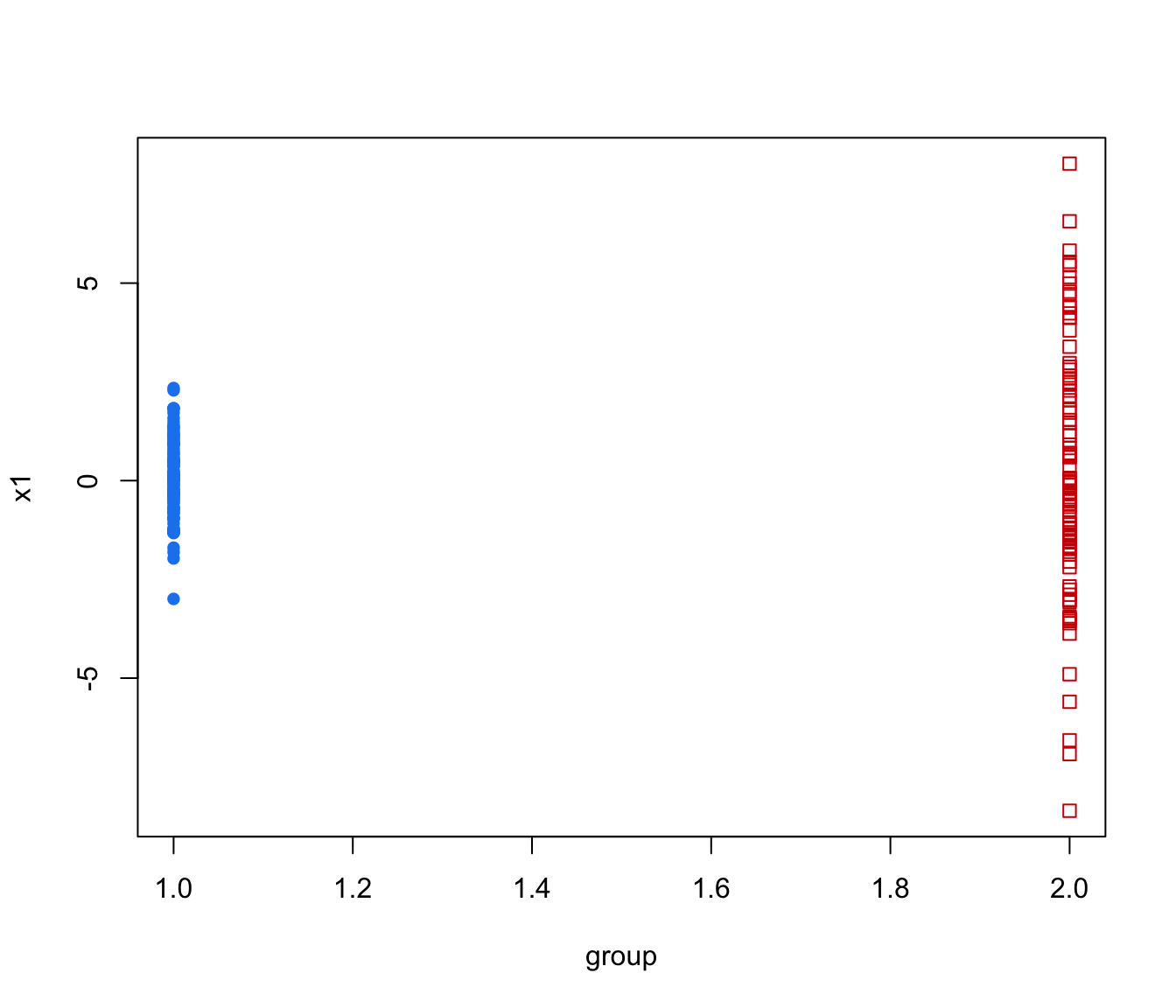Simulate data from a parameterized MVN mixture model.

simE(parameters, n, seed = NULL, ...)
simV(parameters, n, seed = NULL, ...)
simEII(parameters, n, seed = NULL, ...)
simVII(parameters, n, seed = NULL, ...)
simEEI(parameters, n, seed = NULL, ...)
simVEI(parameters, n, seed = NULL, ...)
simEVI(parameters, n, seed = NULL, ...)
simVVI(parameters, n, seed = NULL, ...)
simEEE(parameters, n, seed = NULL, ...)
simVEE(parameters, n, seed = NULL, ...)
simEVE(parameters, n, seed = NULL, ...)
simVVE(parameters, n, seed = NULL, ...)
simEEV(parameters, n, seed = NULL, ...)
simVEV(parameters, n, seed = NULL, ...)
simEVV(parameters, n, seed = NULL, ...)
simVVV(parameters, n, seed = NULL, ...)

Arguments

parameters

A list with the following components:

pro

A vector whose kth component is the mixing proportion for the kth component of the mixture model. If missing, equal proportions are assumed.

mean

The mean for each component. If there is more than one component, this is a matrix whose kth column is the mean of the kth component of the mixture model.

variance

A list of variance parameters for the model. The components of this list depend on the model specification. See the help file for mclustVariance for details.

n

An integer specifying the number of data points to be simulated.

seed

An optional integer argument to set.seed() for reproducible random class assignment. By default the current seed will be used. Reproducibility can also be achieved by calling set.seed before calling sim.

...

Catches unused arguments in indirect or list calls via do.call.

Value

A matrix in which first column is the classification and the remaining columns are the n observations simulated from the specified MVN mixture model.

Attributes:

"modelName" A character string indicating the variance model used for the simulation.

Details

This function can be used with an indirect or list call using do.call, allowing the output of e.g. mstep, em me, Mclust, to be passed directly without the need to specify individual parameters as arguments.

Examples

# \donttest{
d <- 2
G <- 2
scale <- 1
shape <- c(1, 9)

O1 <- diag(2)
O2 <- diag(2)[,c(2,1)]
O <- array(cbind(O1,O2), c(2, 2, 2))
O
#> , , 1
#>
#>      [,1] [,2]
#> [1,]    1    0
#> [2,]    0    1
#>
#> , , 2
#>
#>      [,1] [,2]
#> [1,]    0    1
#> [2,]    1    0
#>

variance <- list(d= d, G = G, scale = scale, shape = shape, orientation = O)
mu <- matrix(0, d, G) ## center at the origin
simdat <- simEEV( n = 200,
parameters = list(pro=c(1,1),mean=mu,variance=variance),
seed = NULL)

cl <- simdat[,1]

sigma <- array(apply(O, 3, function(x,y) crossprod(x*y),
y = sqrt(scale*shape)), c(2,2,2))
paramList <- list(mu = mu, sigma = sigma)
coordProj( simdat, paramList = paramList, classification = cl)
#> Warning: "paramList" is not a graphical parameter
#> Warning: "paramList" is not a graphical parameter
#> Warning: "paramList" is not a graphical parameter
#> Warning: "paramList" is not a graphical parameter
#> Warning: "paramList" is not a graphical parameter
#> Warning: "paramList" is not a graphical parameter# }